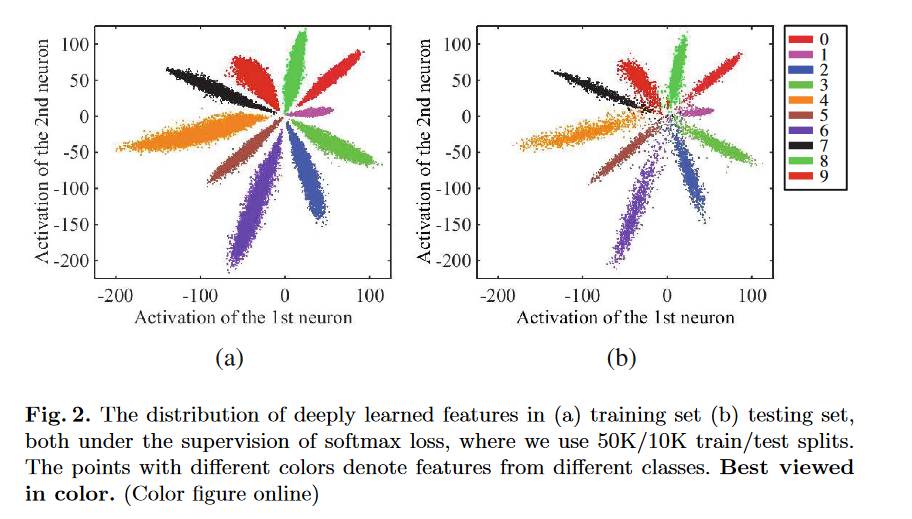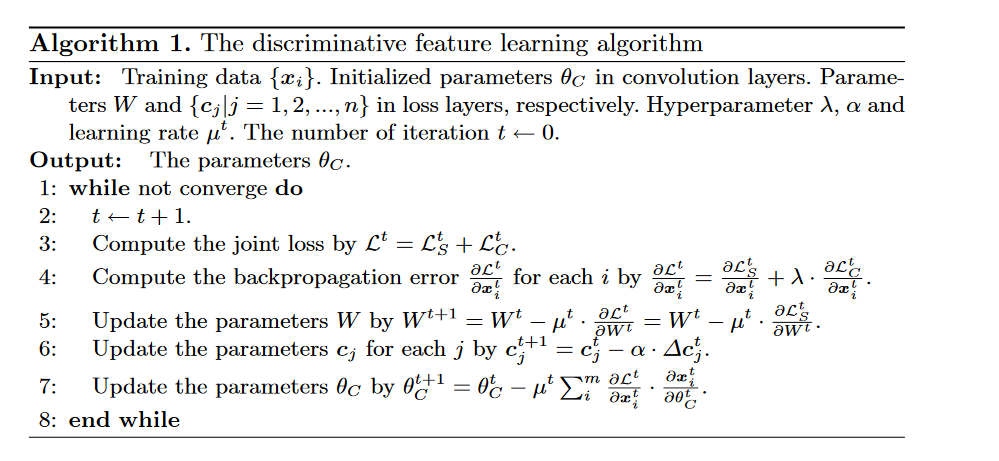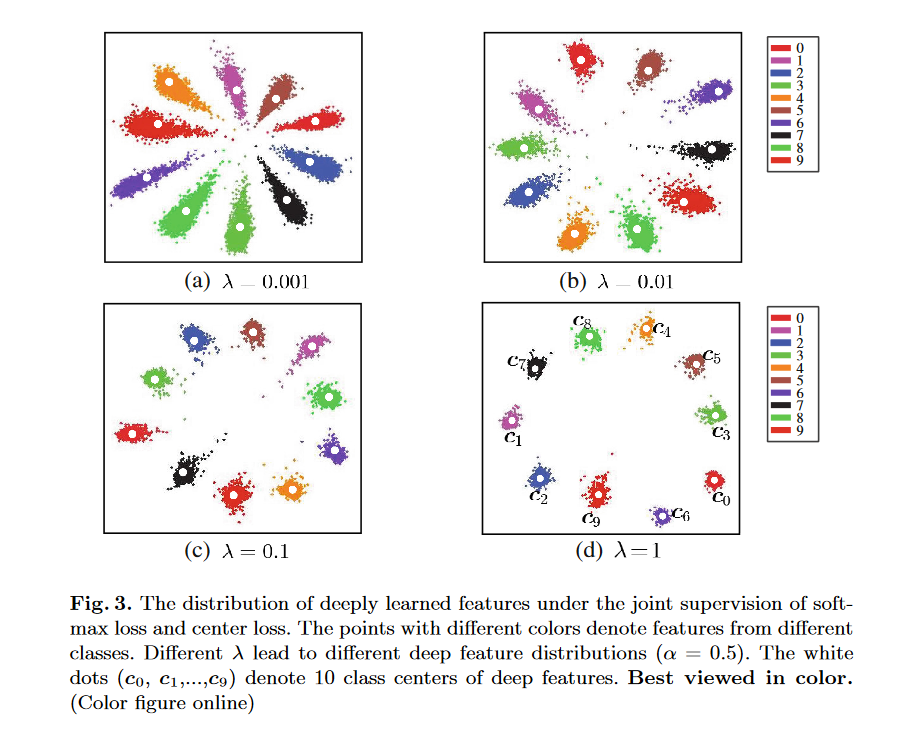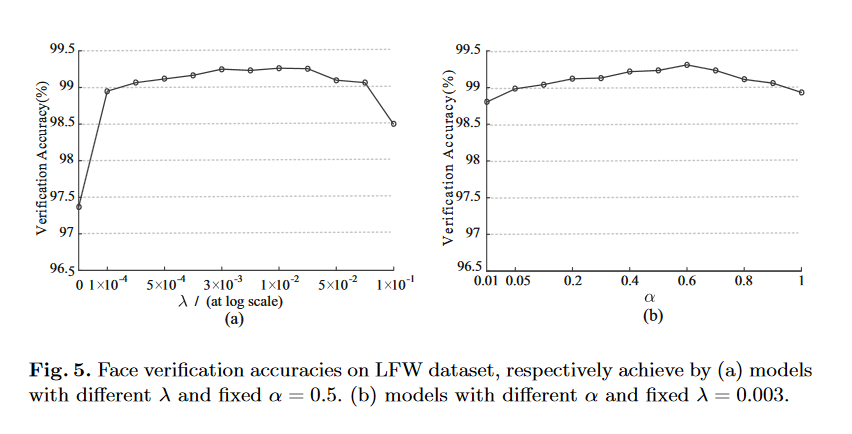# 介绍¶Figure2(a)表示训练集的特征，可以看到不同的类别之间的特征是有明显的间隔的，所以分类器很容易区分样本的类别。而Figure2(b)表示的是测试集的特征，可以看到，有些测试集样本的特征位于分类的边界上，这使得分类器非常难以判断这些样本的具体类别。这种现象用更专业的话来说就是分类器的特征类间相似度小，而类内相似度大。这和聚类里面的思想有一点像，聚类的时候需要尽可能的让同一个簇里面的相似度更大大，而簇之间的相似度更小，这样聚类的结果才更好。

# Center Loss¶

Center Loss可以直接对样本特征之间的距离进行约束。Center Loss添加的约束是，特征与相同类别的平均特征（有"Center"的味道了）的距离要足够小，即要求同类特征要更加接近它们的中心点，用公式来表达：

$L_{C}=\frac{1}{2}\sum_{i=1}^m||x_i-c_{y_i}||_2^2$# 实验结果¶# 代码实现¶

import torch
import torch.nn as nn
from torch.autograd.function import Function

class CenterLoss(nn.Module):
def __init__(self, num_classes, feat_dim, size_average=True):
super(CenterLoss, self).__init__()
self.centers = nn.Parameter(torch.randn(num_classes, feat_dim))
self.centerlossfunc = CenterlossFunc.apply
self.feat_dim = feat_dim
self.size_average = size_average

def forward(self, label, feat):
batch_size = feat.size(0)
feat = feat.view(batch_size, -1)
# To check the dim of centers and features
if feat.size(1) != self.feat_dim:
raise ValueError("Center's dim: {0} should be equal to input feature's \
dim: {1}".format(self.feat_dim,feat.size(1)))
batch_size_tensor = feat.new_empty(1).fill_(batch_size if self.size_average else 1)
loss = self.centerlossfunc(feat, label, self.centers, batch_size_tensor)
return loss

class CenterlossFunc(Function):
@staticmethod
def forward(ctx, feature, label, centers, batch_size):
ctx.save_for_backward(feature, label, centers, batch_size)
centers_batch = centers.index_select(0, label.long())
return (feature - centers_batch).pow(2).sum() / 2.0 / batch_size

@staticmethod
feature, label, centers, batch_size = ctx.saved_tensors
centers_batch = centers.index_select(0, label.long())
diff = centers_batch - feature
# init every iteration
counts = centers.new_ones(centers.size(0))
ones = centers.new_ones(label.size(0))

counts = counts.scatter_add_(0, label.long(), ones)
return - grad_output * diff / batch_size, None, grad_centers / batch_size, None

def main(test_cuda=False):
print('-'*80)
device = torch.device("cuda" if test_cuda else "cpu")
ct = CenterLoss(10,2,size_average=True).to(device)
y = torch.Tensor([0,0,2,1]).to(device)
print (list(ct.parameters()))
out = ct(y,feat)
print(out.item())
out.backward()